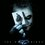# Primitive root

If $n$ is a positive integer, the integers between 1 and $n - 1$ that are coprime to $n$ (or equivalently, the congruence classes coprime to $n$) form a group with multiplication modulo n as the operation; it is denoted by Zn× and is called the group of units modulo n or the group of primitive classes modulo n. As explained in the article multiplicative group of integers modulo n, this group is cyclic if and only if n is equal to 2, 4, p^k, or 2p^k where pk is a power of an odd prime number. A generator of this cyclic group is called a primitive root modulo n, or a primitive element of Zn^×.Note by Sattik Biswas
4 years, 8 months ago

This discussion board is a place to discuss our Daily Challenges and the math and science related to those challenges. Explanations are more than just a solution — they should explain the steps and thinking strategies that you used to obtain the solution. Comments should further the discussion of math and science.

When posting on Brilliant:

• Use the emojis to react to an explanation, whether you're congratulating a job well done , or just really confused .
• Ask specific questions about the challenge or the steps in somebody's explanation. Well-posed questions can add a lot to the discussion, but posting "I don't understand!" doesn't help anyone.
• Try to contribute something new to the discussion, whether it is an extension, generalization or other idea related to the challenge.

MarkdownAppears as
*italics* or _italics_ italics
**bold** or __bold__ bold
- bulleted- list
• bulleted
• list
1. numbered2. list
1. numbered
2. list
Note: you must add a full line of space before and after lists for them to show up correctly
paragraph 1paragraph 2

paragraph 1

paragraph 2

[example link](https://brilliant.org)example link
> This is a quote
This is a quote
    # I indented these lines
# 4 spaces, and now they show
# up as a code block.

print "hello world"
# I indented these lines
# 4 spaces, and now they show
# up as a code block.

print "hello world"
MathAppears as
Remember to wrap math in $$ ... $$ or $ ... $ to ensure proper formatting.
2 \times 3 $2 \times 3$
2^{34} $2^{34}$
a_{i-1} $a_{i-1}$
\frac{2}{3} $\frac{2}{3}$
\sqrt{2} $\sqrt{2}$
\sum_{i=1}^3 $\sum_{i=1}^3$
\sin \theta $\sin \theta$
\boxed{123} $\boxed{123}$

Sort by:

What is the , ,  supposed to mean?

- 4 years, 8 months ago

Sorry it was a mistake, I removed it... by the way root ta ki bhabe likbo goh? LaTex?

$\sqrt{x}$

- 4 years, 8 months ago

You still have  in there. On Brilliant, communicating in English is recommended.

I'll edit your comment to make a $\sqrt{x}$. Click on edit and check that for reference.

- 4 years, 8 months ago

ahhhh....i am sorry again..i will remove it..since its my first note I am making too many mistakes.

- 4 years, 8 months ago

What matters is that you're posting notes, which is great!

- 4 years, 8 months ago

thank you very much. You the inspiration man

- 4 years, 8 months ago